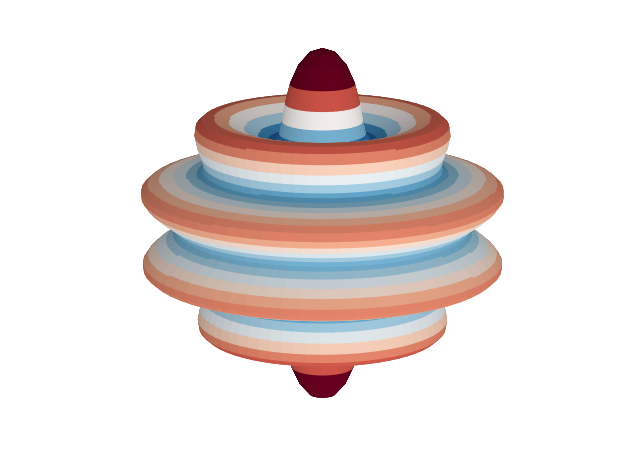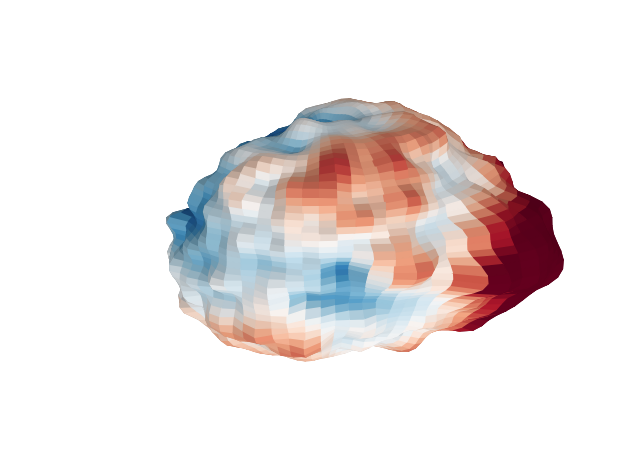# 3D Spherical Harmonic Plots¶

This example demonstrates how to generate a simple 3-dimensional plot of the data in an SHGrid class instance. We start by generating a set of spherical harmonic coefficients that is zero, whith the exception of a single harmonic:

In :
from __future__ import print_function # only necessary if using Python 2.x
%matplotlib inline

import numpy as np
import pyshtools

In :
pyshtools.utils.figstyle(rel_width=0.75)
# %config InlineBackend.figure_format = 'retina'  # if you are using a retina display, uncomment this line

In :
lmax = 30
coeffs = pyshtools.SHCoeffs.from_zeros(lmax)
coeffs.set_coeffs(values=, ls=, ms=)


To plot the data, we first expand it on a grid, and then use the method plot3d():

In :
grid = coeffs.expand()
fig, ax = grid.plot3d(elevation=20, azimuth=30)

/usr/local/lib/python3.7/site-packages/matplotlib/figure.py:457: UserWarning: matplotlib is currently using a non-GUI backend, so cannot show the figure
"matplotlib is currently using a non-GUI backend, "Let's try a somewhat more complicated function. Here we will calculate a random realization of a process whose power spectrum follows a power law with exponent -2:

In :
ldata = 30
degrees = np.arange(ldata+1, dtype=float)
degrees = np.inf

power = degrees**(-2)

coeffs2 = pyshtools.SHCoeffs.from_random(power, seed=12345)
grid2 = coeffs2.expand()
fig, ax = grid2.plot3d(elevation=20, azimuth=30)

/usr/local/lib/python3.7/site-packages/matplotlib/figure.py:457: UserWarning: matplotlib is currently using a non-GUI backend, so cannot show the figure
"matplotlib is currently using a non-GUI backend, "